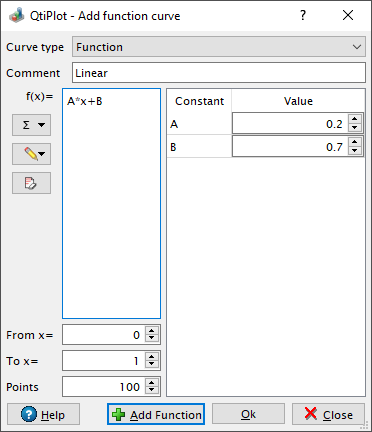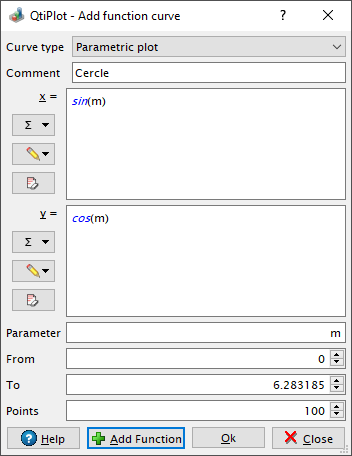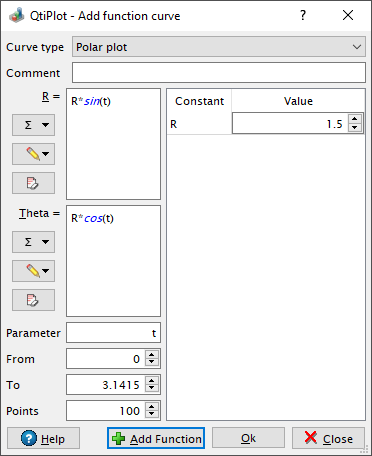Function Dialog

This dialog box is used to add a function curve to the active plot or to edit an already existing function curve. In the latter case it is embedded into the Plot details dialog

The plotted function is built with the common operators *, +, /, -, and ^ (for multiplication, addition, division, subtraction and exponentiation, respectively). A complete set of intrinsic functions are available and these are listed in the muParser section of the chapter on Mathematical Expressions and Scripting.

The first item is the Curve Type and is used to select the form of the generator function. The simplest and most common function definition is the classical Cartesian coordinate definition, y=f(x). This is the default option and is simply named "Function". Just below this is a text pane in which to enter the function definition.

On the left side of the text pane there are two buttons. By pressing the first one, displaying the capital greek letter sigma, a dropdown menu of intrinsic functions grouped by the first letter of their name will be shown. Brief descriptions of the functions are displayed by hovering the cursor over the function name. Selecting a function name will copy it into the function definition pane. By pressing the second button, which displays a pencil icon, a dialog containing a dropdown list of recently typed expressions will pop-up. Selecting an expression will copy it into the function definition pane.

The next two parameters are used to select the X-range to be used for the plot. The third parameter sets the number of data points to be computed in the selected X-range. The Comment input box can be used to enter a text that will be displayed in the plot legend instead of the default function name.

Figure 5-11. The Add Function... dialog box: Cartesian Coordinates.If the function expression contains terms that are recognizable as constants, e.g.: f(x) = a*x + b, QtiPlot will detect them and display a two column table on the right side of the dialog which contains input spin boxes that simplify setting appropriate values for the detected constants.

Figure 5-12. The Add Function... Dialog Box: Automatic Detection of Constants.A function can also be defined using a parametric definition by selecting "Parametric plot" as the Curve type. That is, given some variable, m, the (x,y) data points are computed using functions of that variable, x=f(m) and y=g(m).

The first parameter is the name of the parametric variable (here m). It is followed by the definitions of the two functions, the range of the parametric variable, and the number of data points.

Figure 5-13. The Add Function... dialog box: Parametric Coordinates.Finally, a polar definition of the function may be used by selecting "Polar plot" as the Curve type. Given some variable t, then the radius (R) and angle (Theta) are computed using two functions of that variable, R=f(t) and Theta=g(t), in a manner similar to the parametric function case. The (x,y) data points are then computed as x=R*cos(Theta) and y=R*sin(Theta).

The first parameter is the name of the parametric variable (here t). It is followed by the definition of the two functions, the range of the parametric variable, and the number of data points. Note that the angle is in radians. pi is an internally defined constant which can be used in any mathematical expression. For example, you can use 3*pi to define the parameter range.

Figure 5-14. The Add Function... dialog box: Polar Coordinates.